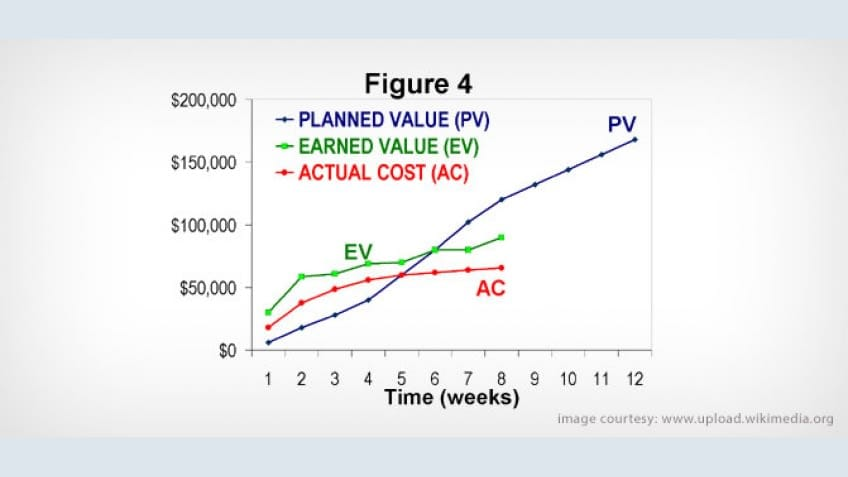## Earned Value Management Part-IV

In the previous articles, I explained the basic concept of Earned Value Management & related formulas which I tried to explain in various different ways. In this part of the article, we shall look at some of the calculations with the help of few examples in detail.

The below examples are used to definitively illustrate how EVM is used beneficially by the PMs. There are numerous more examples available on the net.

Example I

Assume a project that has exactly one task. The task was baselined at 100 hours, but 110 hours have been spent and the estimate to complete is 10 additional hours. The task was to have been completed already. Assume an Hourly Rate of \$100 per hour.

• Hourly Rate = \$100

PV or BCWS = Hourly Rate * Total Hours Planned or Scheduled

PV = \$10000 (\$100 * 100 hours)

• AC or ACWP = Hourly Rate * Total Hours Spent

AC = \$11000 (\$100 * 110 hours) notice actual cost higher than planned cost

• EV or BCWP = Baselined Cost * % Complete Actual

EV = \$9166.667 (baseline of \$10000 * 91.667% complete)

% complete actual is calculated by AC divided by estimated cost at completion which is \$ 11,000 plus cost of 10 additional hours, i.e. \$ 1,000

• BAC = Baselined Effort-hours * Hourly Rate

BAC = \$10,000 (100 hours * \$100) indicates initially budget signed off for the project

• EAC = AC + ETC

EAC = \$12,000 (11,000 + 1,000) notice this is over budget

• VAC = BAC - EAC

VAC = -\$2,000 (\$10,000 - \$12,000) indicates additional funds required to complete work

• % Completed Planned = PV / BAC

% Complete Planned = 100% (\$10,000 PV / \$10,000 BAC)

• % Completed Actual = AC / EAC

% Complete Actual = 91.7% (\$11,000 AC / \$12,000 EAC) lesser than planned completion

• SV = Earned Value (EV) - Planned Value (PV)

SV = -\$833.33 (\$9166.667 EV - \$10,000 PV) negative schedule variance or behind schedule

• SPI = Earned Value (EV) /Planned Value (PV)

SPI = 0.9167 (\$9166.667 EV / \$10,000 PV) indicating poor schedule performance

• CV = Earned Value (EV) - Actual Cost (AC)

CV = -\$1.833.33 (\$9166.667 EV - \$11,000 AC) indicating a cost overrun

• CPI = Earned Value (EV) /Actual Cost (AC)

CPI = 0.833 (\$9166.667 EV / \$11,000 AC) indicating over budget

Example 2

A project has a budget of £10,000,000 and is scheduled for 10 months. It is assumed that the total budget will be spent equally each month until the 10th month is reached. After 2 months the project manager finds that only 5% of the work is finished and a total of £1,000,000 spent.

• PV = £2,000,000 (£10,000,000 divided by 10, multiplied by 2, since 2 months are complete)
• EV = £10,000,000 * 0.05 = £500,000 (Actual cost * % complete)
• AC = £1,000,000
• CV = EV-AC = £500,000 – £1,000,000 = - £500,000
• SV = EV-PV = £500,000 – £2,000,000 = - £1,500,000 or 1.5 months
• CPI = EV/AC = £500,000 / £1,000,000 = 0.5
• SPI = EV/PV = £500,000 / £2,000,000 = 0.25
• EAC = BAC/CPI = £10,000,000 / 0.5 = £20,000,000
• ETC = (BAC-EV) / CPI = (£10,000,000 – £500,000)/ 0.5 = £19,000,000
• Time to compete = (BAC – EV) / CPI = (10,000,000 – 500,000)/0.25 = 38 Months

This project will take total of £20,000,000 and 40 months to complete, twice the cost & four times the schedule estimate indicating significant scope alteration or miscalculation or both.